# Convert Text to Number – Excel & Google Sheets

This tutorial will demonstrate how to convert text to numbers in Excel and Google Sheets.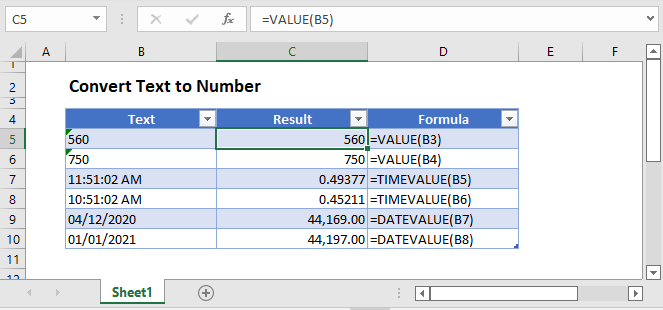## VALUE Function

If you have a number stored as text in Excel, you can convert it to a value by using the VALUE Function.

``=VALUE(B3)``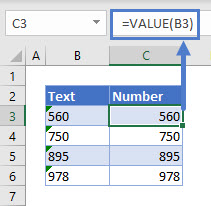## DATEVALUE Function

If you have a date stored as text in Excel, you can get the date value from it by using the DATEVALUE Function.

``=DATEVALUE(B3)``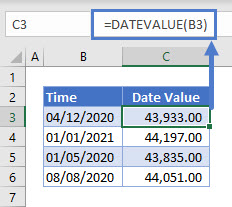This will only work, however, if the date is stored as text.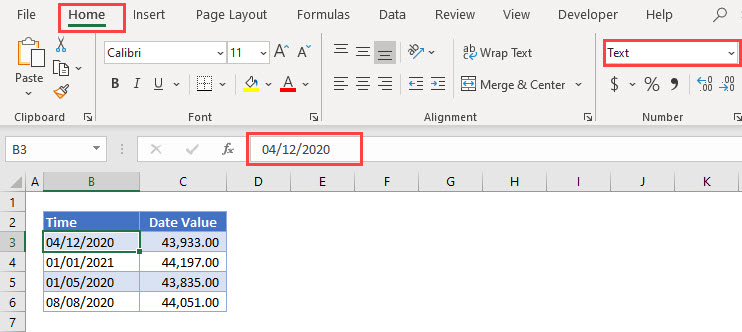If the date is stored as an actual date, the DATEVALUE Function will return an error.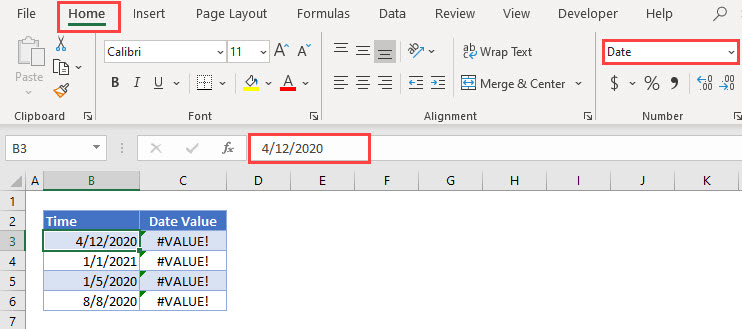## TIMEVALUE Function

If you have time stored as text, you can use the TIMEVALUE Function to return the time value. The TIMEVALUE function will convert the text to an Excel serial number from 0 which is 12:00:00 AM to 0.999988426 which is 11:59.59 PM.

``=TIMEVALUE(B3)``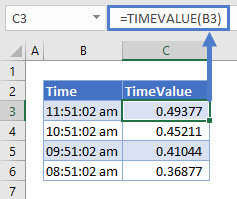## TEXT to COLUMNS

Excel has the ability to convert data stored in a text string into separate columns, using Text to Columns. If there are values within this data, it will convert the value to a number.

1. Select the range to apply the formatting (ex. B3:B6)
2. In the Ribbon, select Data > Text to Columns.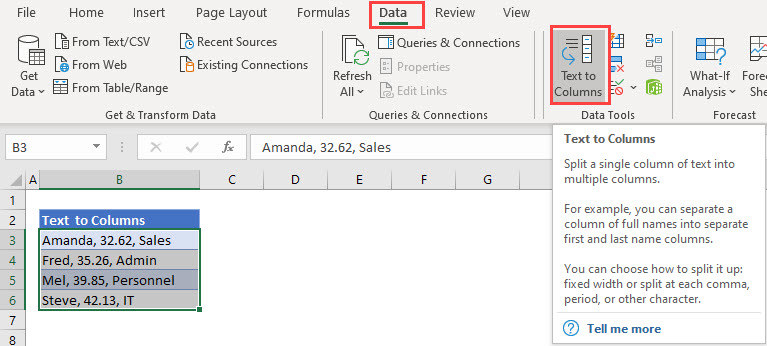1. Keep the option as Delimited, and click Next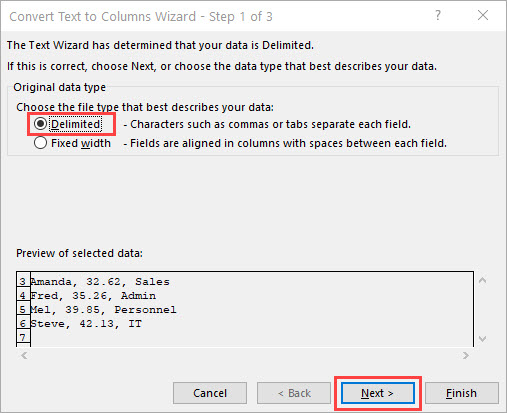1. Select Comma as the Delimiter, and make sure that the Text qualifier is set to {none}.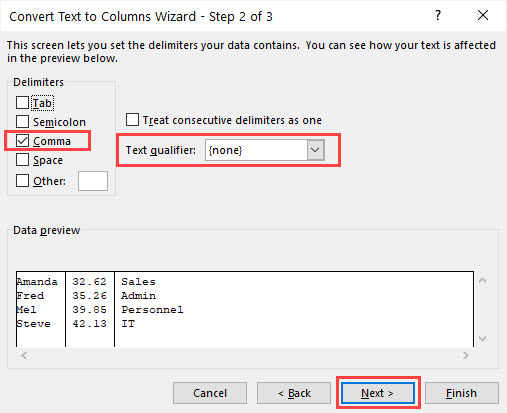1. Select cell C3 as the destination for the data. You do not need to change the Column data format as Excel will find the best match for the data.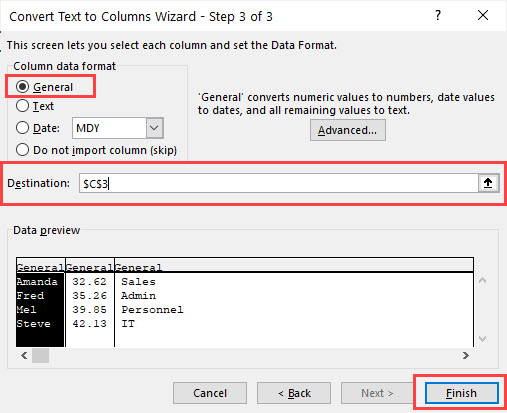1. Click Finish to view the data. If you click in cell D3, you will see that the number part of the text string has been converted to a number.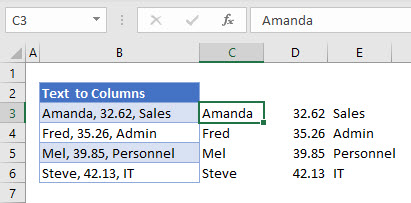## Convert Text to Number in Google Sheets

All of the examples above work the same way in google sheets.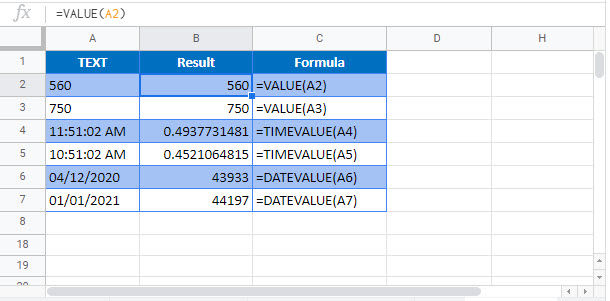### Excel Practice Worksheet

Practice Excel functions and formulas with our 100% free practice worksheets!